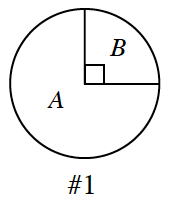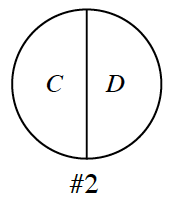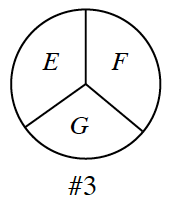### Home > INT2 > Chapter 8 > Lesson 8.4.3 > Problem8-109

8-109.

 Three spinners are shown at right. If each spinner is randomly spun and if spinners #2 and #3 are each equally subdivided, calculate the following probabilities.Use a tree diagram to illustrate all possible combinations that can occur if each spinner is spun once.
Refer to the Math Notes box in Lesson 3.1.4 for information on tree diagrams.

1. P(A, C, and E)

2. P(at least one vowel)

$\frac{5}{6}$